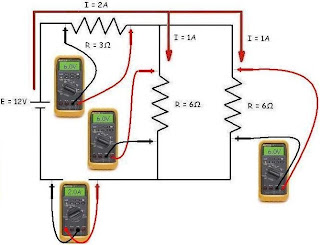## Thursday, June 16, 2011

### Ohm's Law Series-Parallel Circuits Calculation

To end up the discussion of Series-Parallel Circuits, I would like to post this last one remaining topic which is about Ohm's Law of Series-Parallel Circuits for currents and voltages. I did not even mentioned in my previous topics on how to deal with its currents and voltages regarding this type of circuit connection.Ohms Law in Series-Parallel Circuits

Ohm's Law in Series-Parallel Circuits - Current

The total current of the series-parallel circuits depends on the total resistance offered by the circuit when connected across the voltage source. The current flow in the entire circuit and it will divide to flow through parallel branches. In case of parallel branch, the current is inversely proportional to the resistance of the branch - that is the greater current flows through the least resistance and vice-versa. Then, the current will then sum up again after flowing in different circuit branch which is the same as the current source or total current.

The total circuit current is the same at each end of a series-parallel circuit, and is equal to the current flow through the voltage source.

Ohm's Law in Series-Parallel Circuits - Voltage

The voltage drop across a series-parallel circuits also occur the same way as in series and parallel circuits. In series parts of the circuit, the voltage drop depends on the individual values of the resistors. In parallel parts of the circuit, the voltage across each branch are the same and carries a current depends on the individual values of the resistors.

If in case of circuit below, the voltage of the series resistance forming a branch of the parallel circuit will divide the voltage across the parallel circuit. If in case of the single resistance in a parallel branch, the voltage across is the same as the sum of the voltages of the series resistances.﻿
﻿The sum of the voltage across R3 and R4 is the same as the voltage across R2.

Finally, the sum of the voltage drop across each paths between the two terminal of the series-parallel circuit is the same as the total voltage applied to the circuit.

Let's have a very simple example of this calculation for this topic. Considering the circuit below with its given values, lets calculate the total current, current and voltage drop across each resistances.

﻿What is the total current, current and voltage across each resistances
﻿ Here is the simple calculation of the circuit above:

a. Calculate first the total resistance of the circuit:

The equivalent resistance for R2 and R3 is:

R2-3 = 25X50/ 25+50 = 16.67 ohms

R total =  30 ohms + 16.67 ohms = 46.67 ohms

b. Calculate the Total Current using Ohm's Law:

I1 = 120V / 46.67 Ohms = 2.57 Amp. Since R1 is in series connection, the total current is the same for that path.

c. Calculating the voltage drop for R1:

VR1 = 2.57 Amp x 30 ohms = 77.1 volts

d. Calculate the voltage drop across R2 and R3.

Since the equivalent resistance for R2 and R3 as calculated above is 16.67 ohms, we can now calculate the voltage across each branch.

VR2 = VR3 = 2.57 Amp x 16.67 ohms = 42. 84 volts

e. Finally, we can now calculate the individual current for R2 and R3:

I2 = VR2 / R2 = 42.84 volts / 25 ohms =  1.71 Amp.
I3 = VR3 / R3 = 42.84 volts / 50 ohms = 0.86 Amp.

You may also check if the current in each path of the parallel branch are correct by adding its currents:

I1 = I2 + I3 = 1.71 Amp + 0.86 Amp = 2.57 Amp. which is the same as calculated above. Therefore, we can say that our answer is correct.

Cheers!